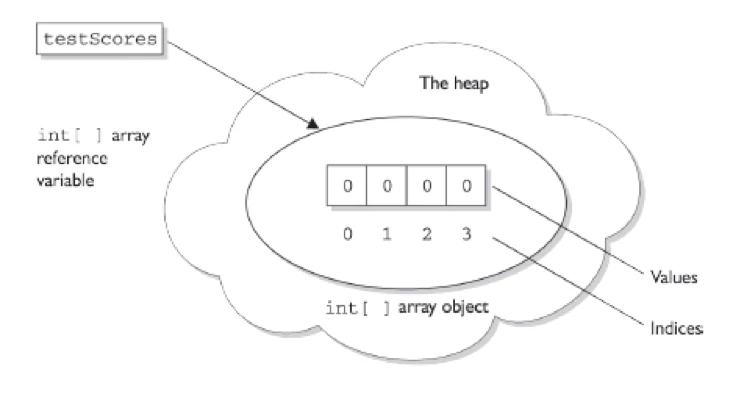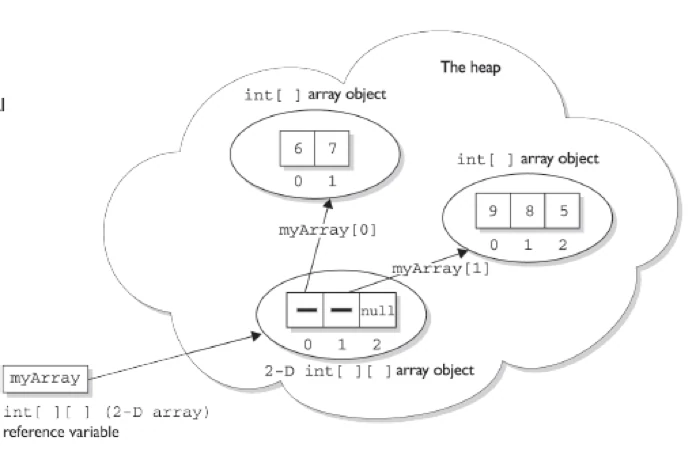Array, what is it? An array is a group of variables of the same data type and referred to by a common name. An array is an object which is a contiguous block of memory locations referred by a common name.

For e.g. To store the marks of 5000 students, you can declare an array, marks, of size 5000 and can store the marks of as many students.

``````int marks[] = new int;
``````

# Need of Arrays

You might come across a situation where you need to store similar type of values for a large number of data items.

For e.g. To store the marks of all the students of a university, you need to declare thousands of variables. In addition, each variable name needs to be unique. To avoid such situations, you can use arrays.

An array consists of a name and the number of elements of the array. You can refer to a specific array element by the array name and the element number, which is known as the index number.

Note: - Array index element number always starts with 0(zero).

# Creating Arrays

The length of an array is fixed at the time of its creation. An array represents related entities having the same data type in contiguous or adjacent memory locations. The related data having data items form a group and are referred to by the same name.

For e.g. employee;

Here, the employee is the name of the array and of size 5. The complete set of values is known as an array and the individual entities are called as elements of the array.

A specific value in an array is accessed by placing the index value of the desired element in a square bracket. Once you create an array, you cannot change its size (although you can, of course, change an individual array element).

• You can refer to a large number of elements by just specifying the index number and the array name.
• Arrays make it easy to do calculations in a loop

The various types of arrays in java are:

• One-dimensional arrays
• Two-dimensional arrays

# Constructing one Dimensional Array

The most straightforward way to construct an array is to use the keyword new followed by the array type, with a bracket specifying how many elements of that type the array will hold. The following is an example of constructing an array of type int:

``````int[] testScores = new int;
``````

The preceding code puts one new object on the heap—an array object holding four elements—with each element containing an int with a default value of 0.So what it does the reference points to a memory location which has been allocated in the memory for the array.

``````int[] EmployeeList = new int[]; // Will not compile; needs a size
``````

Note:- arrays must always be given a size at the time they are constructed. The JVM needs the size to allocate the appropriate space on the heap for the new array object. It is never legal, for example, to do the following:

``````public class Entry {
public static void main(String[] args) {
int[] a = new int;

for(int i=0; i<=4; i++){
a[i] = i;
}

for(int i=0; i<=4; i++){
System.out.println(a[i]);
}
}
}
``````

``````0
1
2
3
4
``````

# Constructing Multidimensional Arrays

Multidimensional arrays, remember, are simply arrays of arrays. So a two dimensional array of type int is really an object of type int array (int []), with each element in that array holding a reference to another int array. The second dimension holds the actual int primitives.

``````int[][] myArray = new int[];
``````Here in this case myArray is pointing to a memory location ( to a array) which in turn refer to other arrays which hold actual values.

``````public class Entry {
// Declare constants
final static int ROWS = 10;

final static int COLS = 5;

public static void main(String[] args) {

// Local varaibles
int rowCount;
int colCount;
int totalSize;

// Declare and allocate an array of bytes
byte[][] screenPix = new byte[ROWS][COLS];

// Obtain and store array dimensions
rowCount = screenPix.length;
colCount = screenPix[COLS].length;
totalSize = rowCount * colCount;

// To obtain the total number of elements of a
// two-dimensional ragged array you need to get the size of
// each array dimension separately

// Display array dimensions
System.out.println("Array row size:    " + rowCount);
System.out.println("Array column size: " + colCount);
System.out.println("Total size:        " + totalSize);

//*************************
//      ragged arrays
//*************************
// First allocate the rows of an array
byte[][] raggedArray = new byte[];

// Now allocate the columns
raggedArray = new byte;
raggedArray = new byte;
raggedArray = new byte;
raggedArray = new byte;
raggedArray = new byte;

// The resulting ragged array is as follows:
//  x x
//  x x
//  x x x x
//  x x x x x x x x
//  x x x

//************************************
//     static array initialization
//************************************
byte[][] smallArray = { { 10, 11, 12, 13 }, { 20, 21, 22, 23 },
{ 30, 31, 32, 33 }, { 40, 41, 42, 43 }, };

// Display the array element at row 2, column 3
System.out.println(smallArray); // Value is 21
}
}

``````

``````Array row size:    10
Array column size: 5
Total size:        50
22
``````

# Anonymous Array

In java it is perfectly legal to create an anonymous array using the following syntax.

``````new <type>[] { <list of values>};
``````

Anonymous array example

``````new int[]{1,2,3};
``````

The above given example creates a nameless array and initializes it. Here, neither name of the array nor the size is specified. It also creates a array which can be assigned to reference or can be passed as a parameter to any method.

``````public class Entry {

public static void main(String[] args) {
System.out.println("Length of array is "
+ Entry.findLength(new int[] { 1, 2, 3 }));
}

public static int findLength(int[] array) {
return array.length;
}
}

``````
``````Length of array is 3
``````

# Assigning values to the Elements of an Array

To access a specific array,

1. You need to specify the name of the array and the index number of the element.
2. The index position of the first element in the array is 0.
``````String designations[];

designations = new String;

designations = "General Manager";

designations= "Managing Director";
``````

# Accessing values

You can access values from elements in the array by referring to the element by its index number.

``````String designations[];

designations = new String;

designations = "General Manager";

designations= "Managing Director”;

designations=designations;
``````

In the above example, the value of the third element of the array is assigned to the first element of the array.